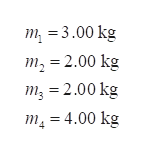# A HW9 - Rotation - PHY201-G, sec xO 1198-MAT127-03: CALCULUSA XMAT 127: Calculus A, sections 0 XBE Ben Eggleston - Grade Calculat xAnswered: An object with a maA webassign.net/web/Student/Assignment-Responses/submit?dep=22685038&tags=autosave#Q7+ Ask Your Teacher5.R My NotesSerPSE10 10.7.OP.021.0/2 pointsPrevious AnswersThe four particles shown below are connected by rigid rods of negligible mass where y, = 6.20 m. The origin is at the center of the rectangle. The system rotates in the xy plane about the zaxis with an angular speed of 5.60 rad/s.2.00 kg3.00 kgУт2.00 kg(4.00 kg4.00 m-(a) Calculate the moment of inertia of the system about the z axis.597,52Note that the distance of the particles from the x axis is 2 6.20 m and the distance from the y axis is ½ 4.00 m. kg • m2(b) Calculate the rotational kinetic energy of the system.9369.11You appear to have calculated the rotational energy correctly using your incorrect result from part (a). JNeed Help?Read ItWatch Iti 12:11

Question
1 views
check_circle

Step 1

The mass of the particles,help_outlineImage Transcriptionclosem, = 3.00 kg m, = 2.00 kg m; = 2.00 kg m, = 4.00 kg fullscreen
Step 2

The distance between the two masses in horizontal direction,

Step 3

The distance between the two mass...

### Want to see the full answer?

See Solution

#### Want to see this answer and more?

Solutions are written by subject experts who are available 24/7. Questions are typically answered within 1 hour.*

See Solution
*Response times may vary by subject and question.
Tagged in

### Physics